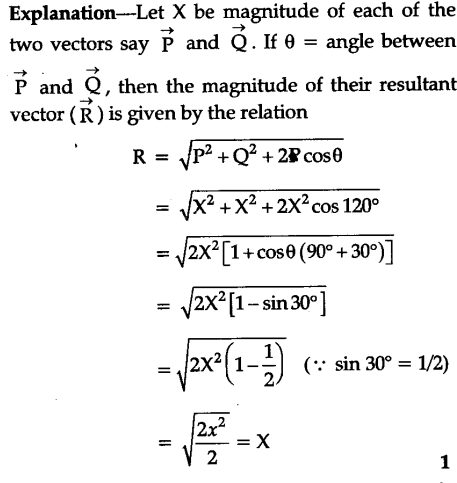# Explain under what conditions, the resultant of two vectors will be equal to either of them

The resultant of two vectors will be equal to either of them if:
(i) The two vectors are of same magnitude.
(ii) The two vectors are inclined to each other at an angle